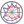Course title
Vector Analysis
 YAMAZAWA HiroshiHIROSE Sampei
Course description
In this lecture we learn vector analysis. A vector analysis is required to put it in the hydrodynamics, the elastic dynamics, the electric magnetic science and the heat conduction theory and develop basic theory as the mathematical means and it has developed. A vector analysis is extension of linear algebra and the differential calculus integration science and is the phenomenon-like contents. I deal with a differential and integral calculus way of vector-valued function in two dimensions or a three-dimensional vector field at this session. It's Stowe camphor tree theorem that the differential calculus and the basic formula which shows that integration is reverse calculation "The definite integration from a in f (x) to b was equal to F (b )- F (a). But, F' (x)= f (x)." were considered in a vector field in the function of 1 variable. This theorem is expansion to 1 of multi variable function of the basic formula of the differential and integral calculus way.
Purpose of class
We learn algebra of vector, the derivative and integration of vector value functions, curves, surface, scalar field and vector field.
Goals and objectives
1. We can find inner product and cross product and calculate the derivative and the integration of vector functions.
2. We can find the length, tangent vector and principal unit normal vector of space curves.
3. We can find the gradient of scalar field, the divergence and curl of vector field and the line integral for vector field.
4. We can explore some example of the divergence theorem and the Stokes theorem.
Language
Japanese
Class schedule

Class schedule HW assignments (Including preparation and review of the class.) Amount of Time Required
1. Vector, inner product text pp.1-5 30minutes
2. Cross product text pp.6-11 30minutes
3. Derivative and integration of vector function text pp.14-20 30minutes
4. Scalar field, gradient text pp.20-27 30minutes
5. divergent, curl text pp.28-35 30minutes
6. space curve text pp.36-39 30minutes
7. scaler's line integrals, vector's line integrals text pp.40-43 30minutes
8. surface, scalar's surface integrals, vector's surface integrals text pp.43-47 30minutes
9. divergence text pp.50-52 30minutes
10. divergence theorem text pp.52-56 30minutes
11. crul text pp.57-59 30minutes
12. Stokes theorem text pp.59-61 30minutes
13. Applications of integral theorems text pp.62-71 30minutes
14. exam lesson 1〜13 30minutes
Total. - - 420minutes
Relationship between 'Goals and Objectives' and 'Course Outcomes'

exercise exam Total.
1. 5% 10% 15%
2. 10% 20% 30%
3. 10% 20% 30%
4. 5% 20% 25%
Total. 30% 70% -
Evaluation method and criteria
Report and exercise 30%, exam 70%, total 100p
Textbooks and reference materials

Prerequisites

Office hours and How to contact professors for questions
• After lecture
Regionally-oriented
Non-regionally-oriented course
Development of social and professional independence
• Course that cultivates an ability for utilizing knowledge
Active-learning course
More than one class is interactive
Course by professor with work experience
Work experience Work experience and relevance to the course content if applicable
N/A N/A
Education related SDGs:the Sustainable Development Goals• 4.QUALITY EDUCATION